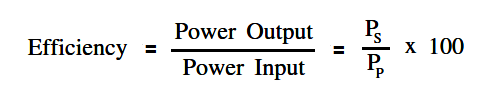# Transformer Efficiency

Efficiency of a transformer is the ratio of the power output to the power input, as illustrated by Equation.where

PS = power of secondary

PP = power of primary